# 基于三维空间关于海豚围捕沙丁鱼群的运动规律Movement Law of Sardines around the Dolphins Based on Three-Dimensional Space

• 全文下载: PDF(3085KB)    PP.299-313   DOI: 10.12677/CSA.2019.92035
• 下载量: 438  浏览量: 955

vkt+1=w*vkt+c1r1(pt-xkt)+c2r2(pg-xkt)

Xkt+1=Xkt+vkt+1

Biologists Craig Reynolds was proposed in 1987 by a very influential birds gather model and thus we can come to the conclusion of the four movement principles of discrete cluster: collision avoid-ance, speed matching, center gather, and inertia factors. In the study, the particle swarm optimization is initialized to a group of random particles, and then we find the optimal solution through iteration; in each iteration, the particles update themselves by tracking two extreme values. There-fore, according to the four movement principles of the cluster, the weights of each direction are obtained, and the data model of the individual motion direction can be set up to update the motion state. Among them, through iteration, we can analyze the characteristics of individual fish swimming, and then promote the influence of individual fish on individual fish movement. Based on the particle swarm optimization algorithm, the initializing model of individual dolphin and sardine group was established to simulate the movement relationship between individual dolphin and sardine group. In the same way, we have consulted the data to develop a strategy for dolphins to round up sardines: in brief, it is very particular about the formation of soldiers. The soldiers are divided into several ways, some of which have a pocket-shaped encirclement. Some of the dolphins act as “catch-ups”, and the prey is Drive away from the circle to prevent the sardines from spreading and fleeing. In this paper, we combine the particle swarm algorithm and the swarm motion model to realize the mating behavior of dolphins. Set up dolphins to search for food in a three-dimensional tar-get space; group size is n; suppose the leader’s position in space is xk ; movement speed is vk. The best food location that the leader found in space is recorded as pg targeting the optimal location for searching for food. Adjust the position Xk(t+1) of the information owner at t + 1 and the direction of motion vk(t+1):

vkt+1=w*vkt+c1r1(pt-xkt)+c2r2(pg-xkt)

Xkt+1=Xkt+vkt+1

According to this, the interaction between dolphins can be analyzed with particle swarm optimization algorithm, and the simulation can be carried out with MATL

1. 引言

1.1. 问题的产生

2. 问题的分析

2.1. 分析集群中单个鱼游动的特点进行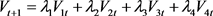2.2. 分析海豚群体与沙丁鱼群体的速度特点

2.3. 合理运用粒子群优化算法来表示海豚个体与沙丁鱼群的运动变化， 多只海豚相互配合捕食沙丁鱼的运动情况，并用MATLAB进行仿真检测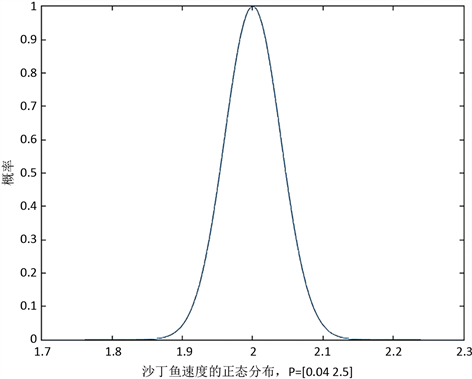Figure 1. Normal distribution of sardine speed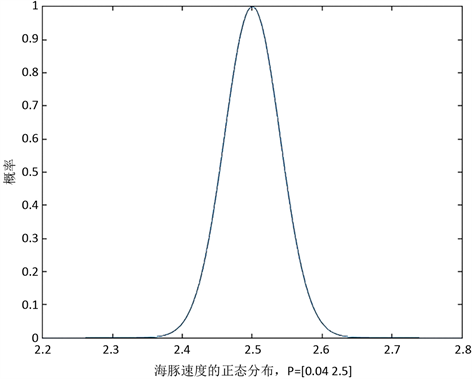Figure 2. Normal distribution of dolphin velocity

1) 避免与邻域个体相冲撞；

2) 匹配邻域个体的速度；

3) 飞向鸟群中心，且整个群体飞向目标。

PSO初始化为一群随机粒子(随机解)，然后通过迭代找到最优解。在每一次迭代中，粒子通过跟踪两个极值来更新自己；第一个就是粒子本身所找到的最优解，这个解称为个体极值；另一个极值是整个种群目前找到的最优解，这个极值是全局极值。另外也可以不用整个种群而只是用其中一部分作为粒子的邻居，那么在所有邻居中的极值就是局部极值。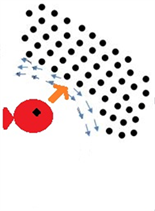(a)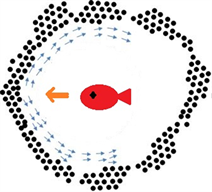(b)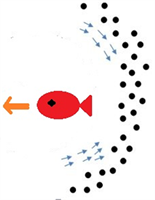(c)

Figure 3. Schematic diagram of the effects of individual dolphins on fish movement: (black spots are sardines)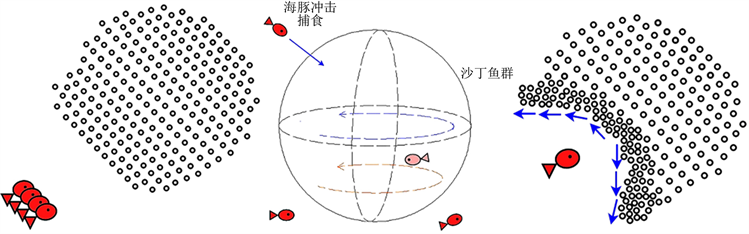(a) (b) (c)

Figure 4. Schematic diagram of the effect of interacting dolphins on fish movement: (black spots are sardines)

3. 模型的建立和求解

3.1. 沙丁鱼运动规则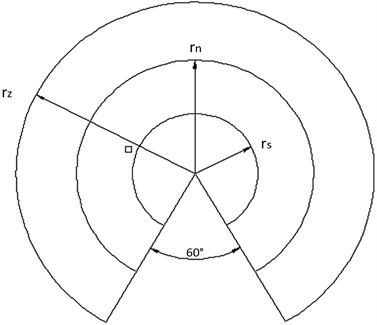Figure 5. Schematic diagram of the range of fish visual capabilities

1) 避免碰撞：避免和相邻个体发生碰撞，当相邻个体进入独立区域时，该沙丁鱼回向相反方向避让。

2) 速度匹配：匹配邻域个体的速度，与整个群体运动的速度几乎一致。

3) 中心聚集：系统中所有个体向中心聚集。

4) 惯性因素：因为个体鱼的游动惯性而对下一周期的运动方向产生的影响。

5) 威胁源：当海豚靠近沙丁鱼，出现在沙丁鱼感知的排外区域范围内时，沙丁鱼将产生避险意识，避险方向为海豚到沙丁鱼的方向。

${V}_{t+1}={V}_{1t}+{V}_{2t}+{V}_{3t}+{V}_{4t}+{V}_{5t}$

${V}_{t+1}={\lambda }_{1}{V}_{1t}+{\lambda }_{2}{V}_{2t}+{\lambda }_{3}{V}_{3t}+{\lambda }_{4}{V}_{4t}+{\lambda }_{5}{V}_{5t}$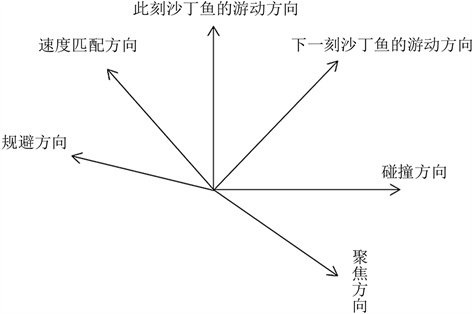Figure 6. The swimming direction of the next sardine

1) 靠近规则的实现：每个个体都有向邻居中心靠拢的特性，邻居中心为观察范围内各个体所在位置的平均值。公式表示为：

$\begin{array}{l}\stackrel{¯}{V}=\frac{\sum {V}_{i}}{N}\left(i\in N\right)\\ {V}_{2t}=\left(\frac{\stackrel{¯}{x}-{x}_{0}}{\sqrt{{\left(\stackrel{¯}{x}-{x}_{0}\right)}^{2}+{\left(\stackrel{¯}{y}-{y}_{0}\right)}^{2}+{\left(\stackrel{¯}{z}-{z}_{0}\right)}^{2}}},\frac{\stackrel{¯}{y}-{y}_{0}}{\sqrt{{\left(\stackrel{¯}{x}-{x}_{0}\right)}^{2}+{\left(\stackrel{¯}{y}-{y}_{0}\right)}^{2}+{\left(\stackrel{¯}{z}-{z}_{0}\right)}^{2}}},\frac{\stackrel{¯}{z}-{z}_{0}}{\sqrt{{\left(\stackrel{¯}{x}-{x}_{0}\right)}^{2}+{\left(\stackrel{¯}{y}-{y}_{0}\right)}^{2}+{\left(\stackrel{¯}{z}-{z}_{0}\right)}^{2}}}\right)\end{array}$

$\stackrel{¯}{V}\left(\stackrel{¯}{x},\stackrel{¯}{y},\stackrel{¯}{z}\right)$ 为邻居的平均值， ${P}_{0}\left({x}_{0},{y}_{0},{z}_{0}\right)$ 为当前个体的位置， ${P}_{i}$ 为当前各个邻居的位置。

2) 对齐规则的实现：沙丁鱼会和它的邻居朝同一个方向游动。公式表示为：

${V}_{3t}=\frac{\sum {V}_{i}}{|N|}\left(i\in N\right)$

${V}_{i}$ 为各个邻居的方向，N为邻居的个数， ${V}_{3t}$ 为邻居的平均方向。

3) 避免碰撞规则的实现：当个体和它的邻居靠的太近时(距离小于碰撞距离)，应自动避开。公式表示为：

$\begin{array}{l}{V}_{4t}=\sum \left(\frac{\left(\stackrel{¯}{x}-{x}_{0}\right)/|M|}{\sqrt{{\left(\stackrel{¯}{x}-{x}_{0}\right)}^{2}+{\left(\stackrel{¯}{y}-{y}_{0}\right)}^{2}+{\left(\stackrel{¯}{z}-{z}_{0}\right)}^{2}}},\frac{\left(\stackrel{¯}{y}-{y}_{0}\right)/|M|}{\sqrt{{\left(\stackrel{¯}{x}-{x}_{0}\right)}^{2}+{\left(\stackrel{¯}{y}-{y}_{0}\right)}^{2}+{\left(\stackrel{¯}{z}-{z}_{0}\right)}^{2}}},\\ \text{\hspace{0.17em}}\text{\hspace{0.17em}}\text{\hspace{0.17em}}\text{\hspace{0.17em}}\text{\hspace{0.17em}}\text{\hspace{0.17em}}\text{\hspace{0.17em}}\text{\hspace{0.17em}}\text{\hspace{0.17em}}\text{\hspace{0.17em}}\text{\hspace{0.17em}}\frac{\left(\stackrel{¯}{z}-{z}_{0}\right)|M|}{\sqrt{{\left(\stackrel{¯}{x}-{x}_{0}\right)}^{2}+{\left(\stackrel{¯}{y}-{y}_{0}\right)}^{2}+{\left(\stackrel{¯}{z}-{z}_{0}\right)}^{2}}}\right)\text{}\left(i\in M\right)\end{array}$

${V}_{4t}$ 为小于碰撞距离的邻居到当前个体方向的平均值，M为邻居中小于碰撞距离的邻居个数。

4) 由于沙丁鱼在运动中有一定的速度，在上一个周期的运动方向对下一周期的运动产生一定的影响。该影响与沙丁鱼的运动速度成正相关，因为假设沙丁鱼速度为常值，此处，惯性影响也假设为常值，用 ${V}_{1t}$ 表示。

5) 结合威胁源的特点，增加了“威胁躲避”对游动方向影响的分析。当海豚靠近沙丁鱼，出现在沙丁鱼的感知范围内时，沙丁鱼将产生避险意识，避险方向为海豚到沙丁鱼的方向，同时向其感知范围内相邻的沙丁鱼发送告警信号，接受到告警信号的沙丁鱼将产生离散意识，离散方向为其感知到的避险沙丁鱼游动方向的平均方向。则此时沙丁鱼的游动方向，公式表示为：

${V}_{5t}=\mathrm{arctan}\frac{{y}_{0}-{y}_{5t}}{{x}_{0}-{x}_{5t}}$

3.2. 模型

3.2.1. 沙丁鱼群运动模型

1、当鱼群处于平衡态时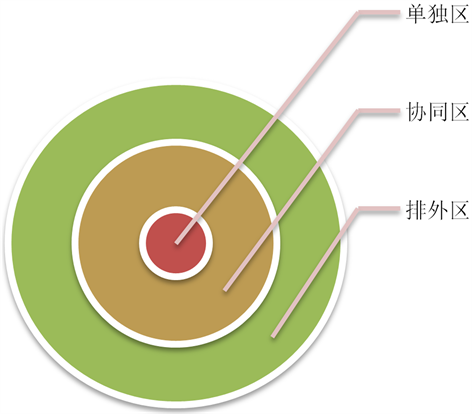Figure 7. Schematic diagram of the internal scope of the population model

2、当鱼群处于非平衡态时(海豚个体对鱼群运动的影响)

1) 建立粒子群

${X}_{i}=\left({x}_{i1},{x}_{i2},\cdots ,{x}_{iD}\right)$ , $i=1,2,3,\cdots ,N$

${V}_{i}=\left({v}_{i1},{v}_{i2},\cdots ,{v}_{iD}\right)$ , $i=1,2,3,\cdots ,N$

${p}_{best}=\left({p}_{i1},{p}_{i2},\cdots ,{p}_{iD}\right)$ , $i=1,2,3,\cdots ,N$

${g}_{best}=\left({p}_{g1},{p}_{g2},\cdots ,{p}_{gD}\right)$ , $i=1,2,3,\cdots ,N$

${v}_{id}=w\ast {v}_{id}+{c}_{1}{r}_{1}\left({p}_{id}-{x}_{id}\right)+{c}_{2}{r}_{2}\left({p}_{gd}-{x}_{id}\right)$

${x}_{id}={x}_{id}+{v}_{id}$

2) 确定算法的流程：

① 初始化粒子群，包括群体规模n，每个粒子的位置 ${x}_{i}$ 和速度 ${v}_{i}$

② 计算每个粒子的适应度值 ${F}_{it}\left[i\right]$

③ 对每个粒子，用它的适应度值 ${F}_{it}\left[i\right]$ 和个体极值 ${p}_{best}\left(i\right)$ 比较，如果 ${F}_{it}\left[i\right]>{p}_{best}\left[i\right]$ ，则用 ${F}_{it}\left[i\right]$ 替换掉 ${p}_{best}\left[i\right]$

④ 对每个粒子，用它的适应度值 ${F}_{it}\left[i\right]$ 和全局极值值 ${g}_{best}$ 比较，如果 ${F}_{it}\left[i\right]>{p}_{best}$ ，则用 ${F}_{it}\left[i\right]$${g}_{best}$

⑤ 根据公式(1)，(2)更新粒子的速度 ${v}_{i}$ 和位置 ${x}_{i}$

⑥ 如果满足结束条件(误差足够好或到达最大循环次数)退出，否则返回②。

3) 粒子群优先算法的数学表达：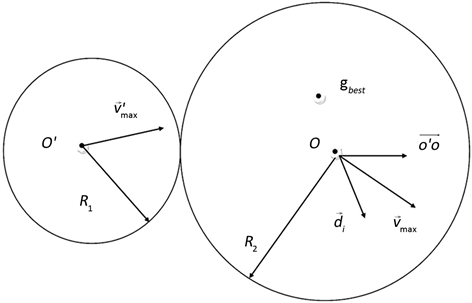Figure 8. Dolphin predation range and sardine escape

$\left\{\begin{array}{l}\stackrel{\to }{{d}_{p}}=\stackrel{¯}{{O}^{\prime }{g}_{best}}\\ {v}_{p}={{v}^{\prime }}_{\mathrm{max}}\end{array}\text{\hspace{0.17em}}\text{\hspace{0.17em}}\text{\hspace{0.17em}}\left({O}^{\prime }O\le {R}_{1}+{R}_{2}\right)$ (3)

$\left\{\begin{array}{l}{v}_{ij}\left(t\right)=w{v}_{ij}\left(t-1\right)+\phi r\left({p}_{fj}\left(j\right),j\right)-{X}_{ij}\left(t-1\right)\text{\hspace{0.17em}}\text{\hspace{0.17em}}\text{\hspace{0.17em}}\text{\hspace{0.17em}}\left({O}^{\prime }O\le {R}_{1}+{R}_{2}\right)\\ {v}_{ij}={v}_{\mathrm{max}}\\ \stackrel{\to }{d}\left(t+\Delta t\right)={O}^{\prime }O+\stackrel{\to }{d\left(t\right)}\text{\hspace{0.17em}}\text{\hspace{0.17em}}\text{\hspace{0.17em}}\text{\hspace{0.17em}}\text{\hspace{0.17em}}\text{\hspace{0.17em}}\text{\hspace{0.17em}}\text{\hspace{0.17em}}\text{\hspace{0.17em}}\text{\hspace{0.17em}}\text{\hspace{0.17em}}\text{\hspace{0.17em}}\text{\hspace{0.17em}}\text{\hspace{0.17em}}\text{\hspace{0.17em}}\text{\hspace{0.17em}}\text{\hspace{0.17em}}\text{\hspace{0.17em}}\text{\hspace{0.17em}}\text{\hspace{0.17em}}\text{\hspace{0.17em}}\text{\hspace{0.17em}}\text{\hspace{0.17em}}\text{\hspace{0.17em}}\text{\hspace{0.17em}}\text{\hspace{0.17em}}\text{\hspace{0.17em}}\text{\hspace{0.17em}}\text{\hspace{0.17em}}\text{\hspace{0.17em}}\text{\hspace{0.17em}}\text{\hspace{0.17em}}\text{\hspace{0.17em}}\text{\hspace{0.17em}}\text{\hspace{0.17em}}\left({O}^{\prime }O\ge {R}_{1}+{R}_{2}\right)\end{array}$ (4)

1、当 ${R}_{1}+{R}_{2}\ge {O}^{\prime }O$

2、当 ${R}_{1}+{R}_{2}\le {O}^{\prime }O$

3.2.2. 相互配合的海豚捕捉沙丁鱼模型(多只海豚对沙丁鱼群的影响)

1、粒子群算法对运动情况的分析

${x}_{k}={\left({x}_{k1},{x}_{k2},{x}_{k3}\right)}^{\text{T}},\text{\hspace{0.17em}}\text{\hspace{0.17em}}k=1,2,3,\cdots ,n$

${v}_{k}={\left({v}_{k1},{v}_{k2},{v}_{k3}\right)}^{\text{T}},\text{\hspace{0.17em}}\text{\hspace{0.17em}}k=1,2,3,\cdots ,n$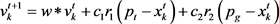${x}_{k}^{t+1}={x}_{k}^{t}+{v}_{k}^{t+1}$

2) ${c}_{1}$${c}_{2}$ 为加速常数。

3) ${r}_{1}$${r}_{2}$ 为两个在[0,1]范围内服从均匀分布的随机变量。

4) 迭代终止条件根据具体问题选为海豚迄今为止搜索到的最优位置满足的预定最小适应阈值。

5) ${c}_{1}$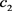和w的取值会直接影响到算法的最终结果。对与感应能力灵敏的海豚， ${c}_{2}$ 和w更是海豚围捕沙丁鱼群运动中不可忽略的部分。

2、海豚的相配合捕食策略

1) 如果沙丁鱼群的数量过多，超过海豚群体的围捕能力，那么海豚将先对沙丁鱼群进行分割。海豚群体从竖直方向将沙丁鱼群切割成围捕能力数量之内的部分，切割完成后两部分沙丁鱼群恢复成球状，海豚向着在围捕能力之内的沙丁鱼群进行围捕。

2) 十分讲究排兵布阵，兵分几路，一部分摆出一个口袋型的包围圈，一部分海豚充当“轰赶者”，将猎物向包围圈中驱赶，防止沙丁鱼扩散逃跑。

3) 由于海豚是哺乳动物，需要经常靠近海面进行换气，所以不能长时间潜入海底。若有n条海豚，其中n − 1条在沙丁鱼群下方防止沙丁鱼群逃向海底，并逐渐使沙丁鱼群向中心靠拢，海豚和沙丁鱼从俯视方向看运动方向都是逆时针；其中1条海豚在沙丁鱼群上部，防止沙丁鱼从上部逃散，同样使沙丁鱼群逐渐聚拢。当鱼群半径r逐渐缩小，沙丁种群密度不断增大，并与n成一定关系的时候，上方的海豚开始捕食沙丁鱼，下方的海豚继续围捕，使鱼群继续聚拢。

4) 当上方海豚吃饱之后，下方某一条海豚开始顶替上方海豚的位置，进行换班捕食。下方海豚继续使沙丁鱼逐渐靠拢，使得海豚群体中每一条海豚都能进行捕食。

3.3. 模型求解(MATLAB图像分析和分析方法)

3.3.1. 具体绘制方法

3.3.2. 沙丁鱼群运动模型(海豚个体对沙丁鱼群运动影响)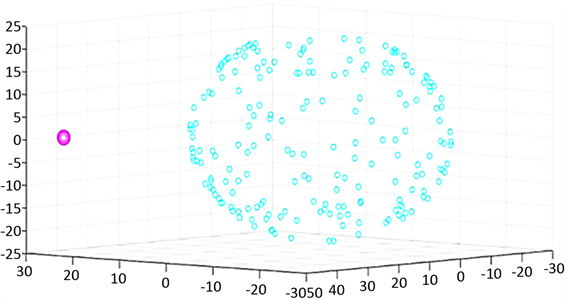Figure 9. Initialization of sardines and dolphins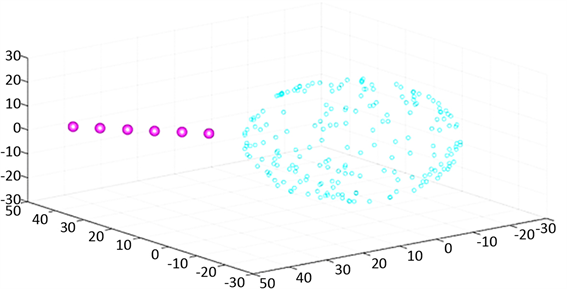Figure 10. Dolphins begin to approach sardines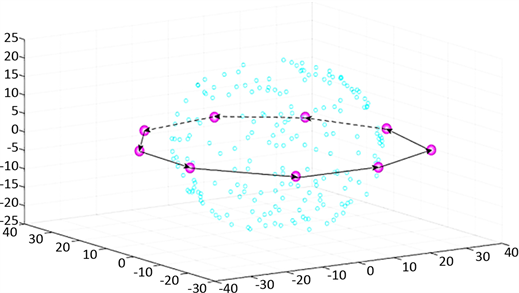Figure 11. Dolphins looking for entry points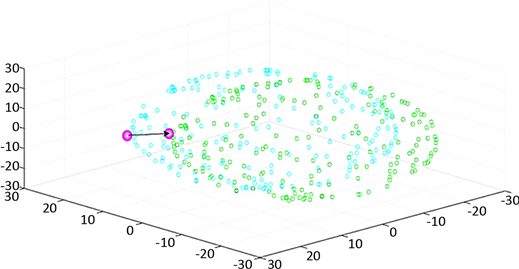Figure 12. The first iterationFigure 13. Repeated multiple times

3.3.3. 相互配合的海豚捕食沙丁鱼模型

1、运动1——海豚接近沙丁鱼群：

2、运动2——海豚对沙丁鱼群进行切割：

4. 模型的评价

4.1. 模型的优点

1) 充分考虑了实际情况，对个体鱼、鱼群行为运动进行了分析和求解。

2) 灵活运用了Matlab等数学软件，进行最模型仿真。

3) 对研究鱼群行为问题提供了借鉴作用。

4) 参考了粒子群算法和鱼群算法的思想，在Vicsek动物群模型和Bold动物群模型的基础上得出了能表示动物群运动的通用模型。通过调整不同作用力的系数大小就可以表示出动物群的觅食、聚群和躲避天敌等运动。具有创新性和通用性。

5) 用几何向量语言描述鲨鱼和沙丁鱼个体的位置变化情况，将鱼群逃逸这一行为数学化、向量化，语言准确科学。整个求解过程中，惯性权重w、加速因子 ${c}_{1}$${c}_{2}$ 和最大速度 ${v}_{\mathrm{max}}$ 共同维护粒子对全局和局部搜索能力的平衡。PSO的一个优势就是采用实数编码，不需要像遗传算法一样采用二进制编码(或者采用针对实数的遗传操作)。粒子具有“记忆”的特性，它们通过“自我”学习和向“他人”学习，使其下一代具有针对性的从“先辈”那里继承更多的信息，从而能在较短的时间内找到最优解。粒子群优化算法的信息共享机制是很不同的，在粒子群优化算法中，信息流动是单向的，即只有 ${g}_{best}$ 将信息给其他的粒子，这使得整个搜索更新过程跟随当前解。

6) 由海豚个体推广到多只海豚配合，优化性更强。

7) 建立的模型由个体到整体，层层递进，条理清晰，便于理解。

4.2. 模型的缺点

1) 文章将动物群的信息传递机制体现在吸引力、排斥力和一致力三个方面，讨论还不够深入问题一加入惯性运动和非惯性运动，使得模型过于细化。

2) 模型中参数的调整主观性较强。

3) 未对沙丁鱼与海豚的交互空间进行严密的分析。

4) 对于一些问题的研究不够专业。

5) 单单考虑距离的影响进行迭代，忽略了水流，光线等因素的干扰。

6) 由于PSO中粒子向自身历史最佳位置和邻域或群体历史最佳位置聚集，形成粒子种群的快速趋同效应，容易出现陷入局部极值、早熟收敛或停滞现象。同时，PSO的性能也依赖于算法参数。

7) 实验假设比较理想，比如将沙丁鱼和海豚都设置成了点来进行模拟仿真。

8) 实验数据都是经过资料查询进行假设的，与现实中的海豚围捕沙丁鱼群还是有一定的差距。

  程淑红. 基于视觉感知的鱼群运动行为特征参数提取[J]. 计量学报, 2017, 38(2): 175-178.  周应祺. 鱼类集群行为的研究进展[J]. 上海海洋大学学报, 2013, 22(5): 734-743.